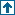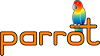parrotcode: Bison specification for the PIR assembly language parser. Contents | Compilers

# NAMEpir.y - Bison specification for the PIR assembly language parser.

# DESCRIPTIONThis file implements the parser for the PIR assembly language. During the parsing phase, data structures are created that represent the input. These data structures are defined in `pircompunit.h`.

The parser implements strength reduction and constant folding. Strength reduction refers to the selection of instructions that have the same effect as the instruction written by the PIR programmer, but are more efficient. For instance:

` add \$P0, \$P0, \$P1`

can be reduced to:

` add \$P0, \$P1`

as `\$P0` was an IN/OUT operand.

Constant folding refers to the compile-time evaluation of expressions, if possible. For instance:

` add \$I0, 10, 20`

can be written as:

` set \$I0, 30`

as we can evaluate this result during compile time. Likewise, conditional branch instructions may become unconditional branch instructions (if the condition evaluates to true during compile time) or it may become a `noop` (no op) instruction (if the condition evaluates to false during compile time).

# FUNCTIONS`static constant *fold_i_i(yyscan_t yyscanner, int a, pir_math_operator op, int b)`

Evaluates the expression `a op b` and returns a constant node containing the result value. Both `a` and `b` are integer values.

`static constant *fold_n_i(yyscan_t yyscanner, double a, pir_math_operator op, int b)`

Same as `fold_i_i`, except `a` is of type double.

`static constant *fold_i_n(yyscan_t yyscanner, int a, pir_math_operator op, double b)`

Same as `fold_i_i`, except `b` is of type double.

`static constant *fold_n_n(yyscan_t yyscanner, double a, pir_math_operator op, double b)`

Same as `fold_i_i`, except that both `a` and `b` are of type double.

`static constant *fold_s_s(yyscan_t yyscanner, char *a, pir_math_operator op, char *b)`

Evaluate the expression `a op b`, where both `a` and `b` are strings. Only the concatenation and comparison operators are implemented; other operators will result in an error.

`static int evaluate_i_i(int a, pir_rel_operator op, double b)`

Compare `a` with `b` according to the relational operator `op`. Wrapper for `evaluate_n_n`, which takes arguments of type double.

`static int evaluate_n_i(int a, pir_rel_operator op, double b)`

Compare `a` with `b` according to the relational operator `op`. Wrapper for `evaluate_n_n`, which takes arguments of type double.

`static int evaluate_i_n(int a, pir_rel_operator op, double b)`

Compare `a` with `b` according to the relational operator `op`. Wrapper for `evaluate_n_n`, which takes arguments of type double.

`static int evaluate_n_n(double a, pir_rel_operator op, double b)`

Compare `a` with `b` according to the relational operator `op`. `op` can be `<!=`>, `<==`>, `<`, `<=`, `>` or `>=`.

`static int evaluate_s_s(char *a, pir_rel_operator op, char *b)`

Compare string `a` with string `b` using the operator `op`. The function uses C's `strcmp` function. Based on that result, which can be -1 (smaller), 0 (equal) or 1 (larger), a boolean result is returned. Note that `strcmp()` should not be replaced by the `STREQ` macro used throughout Parrot source code; this function uses the result of `strcmp()`.

`static int evaluate_s(char *const s)`

Evaluate a string in boolean context; if the string's length is 0, it's false. If the string equals "0", ".0", "0." or "0.0", it's false. Otherwise, it's true.

`static int evaluate_c(lexer_state *const lexer, constant *const c)`

Evaluate a constant node in boolean context; if the constant is numeric, it must be non-zero to be true; if it's a string, `evaluate_s` is invoked to evaluate the string.

`static char *concat_strings(lexer_state *const lexer, char const *a, char const *b)`

Concatenates two strings into a new buffer. The new string is returned.

`static void create_if_instr(yyscan_t yyscanner, lexer_state *lexer, int invert, int hasnull, char *const name, char *const label)`

Create an `if` or `unless` instruction; if `invert` is non-zero (true), the `if` instruction is inverted, effectively becoming `unless`.

If `hasnull` is non-zero (true), the `if` instruction becomes `if_null`; again, if `invert` is non-zero, the instruction becomes `unless_null`.

`name` is the name of the variable that is checked during this instruction

`static int check_value(constant *const c, int val)`

Check whether the current value of the constant `c` equals `val`. For our purposes, it is sufficient to check for integer values (including a check against 1.0 or 0.0). If the values are indeed equal, true is returned, false otherwise. If the constant is not numeric, it returns always false.

`static void reduce_strength(yyscan_t yyscanner, int newop, int op2_index)`

Do the actual strength reduction; the current op will be replaced by `newop`. The operands at position 1 and `op2_index` will be retrieved. `op2_index` indicates the position of the second operand that must be retrieved.

When the current instruction is:

` add_i_i_ic`
then `op2_index` will be 1, indicating the second operand must be retrieved. When the current instruction is:

` add_i_ic_i`
then `op2_index` will be 2, so that the two operands represented by target nodes are retrieved (the operands indicated as `i`, as opposed by `ic`.)

Then, if the two operands (which are target nodes) are equal, then one of them can be removed, so that the direction of the first operand will change from OUT to INOUT.

`static int convert_3_to_2_args(int opcode, int *second_op_index)`

Given the 3-operand version of a Parrot math op (in the parameter `opcode`), get the strength-reduced version with 2 operands. This is a low-level, "dirty-job-but-someone-has-to-do-it" function, so other higher level functions don't get cluttered. If a 2-operand version is specified, then that version is returned.

The second parameter `second_op_index` will be assigned the index of the second target parameter, if any (note this is an out parameter, as it is passed by address). So, in case of `PARROT_OP_add_i_ic_i`, this will be 2, as that's the second target (start counting from 0). In case of `PARROT_OP_add_i_i_ic`, it's 1.

`static void do_strength_reduction(yyscan_t yyscanner)`

Implement strength reduction for the math operators `add`, `sub`, `mul`, `div` and `fdiv`. If the current instruction is any of these, then the first two operands are checked; if both are targets and are equal, the second operand is removed; this means that the first operand will be an IN/OUT operand. For instance:

` add \$I0, \$I0, \$I1`
becomes:

` add \$I0, \$I1`
and

` add \$I0, 1`
becomes:

` inc \$I0`
`static void check_first_arg_direction(yyscan_t yyscanner, char *const opname)`

This function checks the first argument's direction of the op `opname`. If the direction is not `OUT`, a syntax error is emitted. This function assumes that `opname` is a valid parrot op. This check is done to complain about valid PIR syntax that is undesirable, such as:

` \$S0 = print`
which is another way of writing:

` print \$S0`
As the first argument `\$S0` is an `IN` argument, the sugared version should not be allowed.

`static int get_signature_length(expression *const e)`

Calculate the length of the signature for one operand; an operand is separated from the instruction name or another operand through '_', which must also be counted.

` set \$I0, 42  --> set_i_ic`
therefore, for \$I0 (a target), return 1 for the type, 1 for the '_', and whatever is needed for a key, if any, as in this example:

` set \$P0 = "hi"  --> set_p_kic_sc`
\$P0 is a target, resulting in "_p", the key  is a key ('k') of type int ('i'), and it's a constant ('c'). Add 1 for the '_'.

`static char *write_signature(expression *const iter, char *instr_writer)`

Write the signature for the operand `iter`, using the character pointer `instr_writer`. When the operand is an indexed target node (in other words, it has a key node), this function is invoked recursively passing the key as an argument.

This function returns the updated character pointer (due to pass-by-value semantics of the C calling conventions).

`static char *get_signatured_opname(instruction *const instr)`

Returns the full opname of the instruction `name`; the signature of the opname is based on the operands, some examples are shown below:

``` set I0, 10        --> set_i_ic
print "hi"        --> print_sc
set P0, 3.14   --> set_p_kic_nc```
For each operand, an underscore is added; then for the types int, num, string or pmc, an 'i', 'n', 's' or 'p' is added respectively. If the operand is a constant, a 'c' suffic is added.

If the operand is a key of something, a 'k' prefix is added.

`static int get_opinfo(yyscan_t yyscanner)`

Compute the signatured opname from the instruction name and its arguments. Based on this signature, the opcode is retrieved. If the opcode cannot be found (i.e., it's -1), then a check is done for some special math ops; `add_i_ic_ic` and the like do not exist, but instead should be replaced by `set_i_ic` (and the like). If it's not one of these special cases, then that means the op is not valid, and an error message will be reported.

`static void check_op_args_for_symbols(yyscan_t yyscanner)`

Check the arguments of the current instruction. First, the number of expected arguments is checked against the specified number of arguments. Then, for each argument, if the particular argument should not be a label (instructions can take LABEL operands), and if the argument is a target node, then the argument must be a declared symbol. If it is not, an error message is given.

If there are errors, FALSE is returned; if successful, TRUE is returned.